Courses

# NCERT Exemplar (Part - 1) - Gravitation Notes | EduRev

## JEE : NCERT Exemplar (Part - 1) - Gravitation Notes | EduRev

The document NCERT Exemplar (Part - 1) - Gravitation Notes | EduRev is a part of the JEE Course NEET Revision Notes.
All you need of JEE at this link: JEE

MULTIPLE CHOICE QUESTION I

Q.1. The earth is an approximate sphere. If the interior contained matter which is not of the same density everywhere, then on the surface of the earth, the acceleration due to gravity
(a) Will be directed towards the centre but not the same everywhere.
(b) Will have the same value everywhere but not directed towards the centre.
(c) Will be same everywhere in magnitude directed towards the centre.
(d) Cannot be zero at any point.
Ans.
(d)
Solution.
If the density of the earth is non-uniform then ‘g’ at different point on the surface of the earth will be different.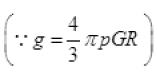If we substitute any value of density, g ≠ 0 at any point.

Q.2. As observed from earth, the sun appears to move in an approximate circular orbit. For the motion of another planet like mercury as observed from earth, this would
(a) Be similarly true.
(b) Not be true because the force between earth and mercury is not inverse square law.
(c) Not be true because the major gravitational force on mercury is due to sun.
(d) Not be true because mercury is influenced by forces other than gravitational forces.
Ans.
(c)
Solution.
Force of attraction between any two objects obeys the inverse square law as its universal law. The relative motion between Earth, Mercury as observed from Earth will not be circular as the force on Mercury due to the sun is very large than due to Earth and due to the relative motion of Sun and Earth with Mercury.

Q.3. Different points in earth are at slightly different distances from the sun and hence experience different forces due to gravitation.
For a rigid body, we know that if various forces act at various points in it, the resultant motion is as if a net force acts on the c.m. (centre of mass) causing translation and a net torque at the c.m. causing rotation around an axis through the c.m.
For the earth-sun system (approximating the earth as a uniform density sphere)
(a) The torque is zero.
(b) The torque causes the earth to spin.
(c) The rigid body result is not applicable since the earth is not even approximately a rigid body.
(d) The torque causes the earth to move around the sun.
Ans.
(a)
Solution.
The torque on earth due to gravitational attractive force on earth is zero, because the direction of force (F) (gravitational) and the line joining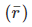, the point of application of force (which also is at c.m. of earth) is along the same direction, so angle between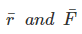is zero or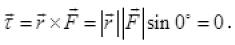Q.4. Satellites orbiting the earth have finite life and sometimes debris of satellites fall to the earth. This is because,
(a) The solar cells and batteries in satellites run out.
(b) The laws of gravitation predict a trajectory spiralling inwards.
(c) Of viscous forces causing the speed of satellite and hence height to gradually decrease.
(d) Of collisions with other satellites.
Ans.
(c)
Solution.
We know that the total energy of the earth satellite of radius R bounded system is negative
(-GM\ 2 R)
where M is mass of the earth. –
Due to the atmospheric friction (viscous force) acting on satellite, energy decreases continuously, radius of the orbit or height decreases gradually and the satellite spirals down with increasing speed till it bums in the denser layers of the atmosphere.

Q.5. Both earth and moon are subject to the gravitational force of the sun. As observed from the sun, the orbit of the moon
(a) Will be elliptical.
(b) Will not be strictly elliptical because the total gravitational force on it is not central.
(c) Is not elliptical but will necessarily be a closed curve.
(d) Deviates considerably from being elliptical due to influence of planets other than earth.
Ans.
(b)
Solution.
Moon revolves around the earth in a nearly circular orbit. When it is observed from the sun, two types of forces are acting on the moon one is due to gravitational attraction between the sun and the moon and the other is due to gravitational attraction between the earth and the moon. So moon is moving under the combined gravitational pull acting on it due to the earth and the sun. Hence, total force on the moon is not central.

Q.6 In our solar system, the inter-planetary region has chunks of matter (much smaller in size compared to planets) called asteroids. They
(a) Will not move around the sun since they have very small masses compared to sun.
(b) Will move in an irregular way because of their small masses and will drift away into outer space.
(c) Will move around the sun in closed orbits but not obey Kepler’s laws.
(d) Will move in orbits like planets and obey Kepler’s laws.
Ans.
(d)
Solution.
Like any other planet, asteroids also follow Kepler’s law and move in elliptical orbits around planet due to the central gravitational force acting on them.
small mass of asteroid does not escape the gravitational force due to sun because of large mass of sun and hence, (a) is incorrect. Since gravitational force follow inverse square law, the asteroid will move in circular or elliptical orbits and (b) is incorrect option

Q.7 Choose the wrong option.
(a) Inertial mass is a measure of difficulty of accelerating a body by an external force whereas the gravitational mass is relevant in determining the gravitational force on it by an external mass.
(b) That the gravitational mass and inertial mass are equal is an experimental result.
(c) That the acceleration due to gravity on earth is the same for all bodies is due to the equality of gravitational mass and inertial mass.
(d) Gravitational mass of a particle like proton can depend on the presence of neighbouring heavy objects but the inertial mass cannot.
Ans.
(d)
Solution.
Inertial mass:
The mass of an object A can be defined as the mass of the object obtained by measuring their accelerations under equal forces taking mass of B to be 1kg. This is known as inertial mass.
Gravitational mass:
The gravitational force exerted by a massive body on an object is proportional to the mass of the object. If FA and FB are the forces of attraction on the two objects due to the earth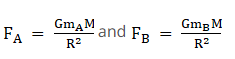Thus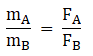If B is a standard unit mass, by measuring gravitational Force we can obtain the mass of object A which is known as gravitational mass.
By several experiments, it has been concluded that the inertial and gravitational mass are identical.

Q.8. Particles of masses 2M, m and M are respectively at points A, B and C with AB = ½ (BC). m is much-much smaller than M and at time t = 0, they are all at rest (Figure).
At subsequent times before any collision takes place: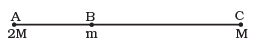(a) m will remain at rest
(b) m will move towards M
(c) m will move towards 2M
(d) m will have oscillatory motion
Ans.
(c)
Solution.
Let FBC be the force experienced by mass m at point B due to mass M at point C, and FBA be the force experienced by mass m at point B due to mass 2M at point A.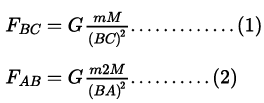Suppose AB = x, then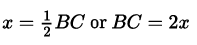Substituting the values of AB and BC in the above equations (1) and (2)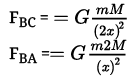As FBA > FBC, m will move towards point A (position of particle with mass 2M).

MULTIPLE CHOICE QUESTIONS II

Q.9 Which of the following options are correct?
(a) Acceleration due to gravity decreases with increasing altitude.
(b) Acceleration due to gravity increases with increasing depth (assume the earth to be a sphere of uniform density).
(c) Acceleration due to gravity increases with increasing latitude.
(d) Acceleration due to gravity is independent of the mass of the earth
Ans.
(a, c)
Solution.
(a) The acceleration due to gravity at an altitude (height),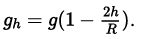increasing height (h) decreases the value of gh. option (a) is correct.
(b) Assuming the earth to be a sphere of uniform density, the acceleration due to gravity at a particular depth (d)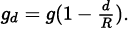Increasing depth (d) decreases the value of gd. Option (b) is incorrect.
(c) If λ is latitude on earth then gλ = g - ω2R cosλ
As value of cos λ decrease from 0º to 90º ( from 1 to 0). The acceleration due to gravity increases from equator (λ = 0º) to pole (λ = 90º). Option (c) is correct.
(d) The acceleration due to gravity on surface of earth is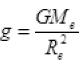So g on earth depends on mass of earth. Option (d) is incorrect.

Q.10 If the law of gravitation, instead of being inverse-square law, becomes an inverse-cube law
(a) planets will not have elliptic orbits.
(b) circular orbits of planets is not possible.
(c) projectile motion of a stone thrown by hand on the surface of the earth will be approximately parabolic.
(d) there will be no gravitational force inside a spherical shell of uniform density.
Ans.
(a, b, c)
Solution.
If the law of gravitation becomes an inverse cube law instead of inverse square law, then for a planet of mass m revolving around the sun of mass M, we can write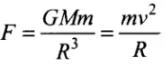(where R is the radius of orbiting planet)
⇒ Orbital Speed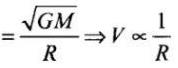Time period of revolution of a planet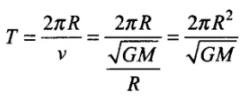⇒ T2 ∝ R4
Hence, orbit will not be elliptical.
[for elliptical orbit T2 ∝ R3]
The circular orbits of the planets is not possible according to new law of gravitation.
As force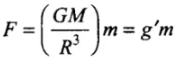where,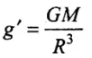As g' acceleration due to gravity is constant, hence path followed by a projectile will be approximately parabolic, (as T ∝ R2).
Also, gravitational force inside a spherical shell of uniform density will have some value. So, only option (d) is incorrect.

Q.11. If the mass of sun were ten times smaller and gravitational constant G were ten times larger in magnitudes
(a) Walking on ground would became more difficult.
(b) The acceleration due to gravity on earth will not change.
(c) Raindrops will fall much faster.
(d) Airplanes will have to travel much faster
Ans.
(a, c, d)
Solution.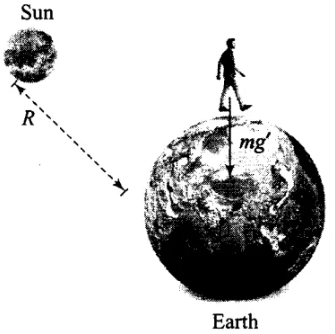According to the problem
G' = 10G
Gravitational field due to the earth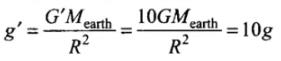Weight of person = mg' = m x 10 g = 10 mg
force on the man due to the sun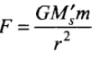As r »R (radius of the earth)
⇒ F will be very small.
So, the effect of the sun will be neglected.
Due to this reason gravity pull on the person will increase. Due to it, walking on ground would become more difficult.
Critical velocity, vc is proportional to g, i.e., vcg
As, g’ > g => vc‘>vc
Hence, rain drops will fall much faster.
To overcome the increased gravitational force of the earth, the aeroplanes will have to travel much faster.

Q.12. If the sun and the planets carried huge amounts of opposite charges,
(a) All three of Kepler’s laws would still be valid
(b) Only the third law will be valid
(c) The second law will not change
(d) The first law will still be valid
Ans.
(a, c, d)
Solution.
Coulomb’s electric force or Electrostatic force of attraction will produce due to opposite charges.
If the sun and the planets carries huge amount of opposite charges, then electrostatic force of attraction will be large. Gravitational force is also attractive in nature have both forces will be added and both are radial in nature.
Both the forces obey inverse square law and are central forces. As both the forces are of same nature, hence all the three Kepler’s laws will be valid.

Q.13. There have been suggestions that the value of the gravitational constant G becomes smaller when considered over very large time period (in billions of years) in the future. If that happens, for our earth,
(a) Nothing will change
(b) We will become hotter after billions of years
(c) We will be going around but not strictly in closed orbits
(d) After sufficiently long time we will leave the solar system
Ans.
(c, d)
Solution. We know that gravitational force exists between the earth and the sun.
FG = G(MS x me)/ r2
Where MS is mass of the sun and me is mass of the earth.
This provides the necessary centripetal force for the circular orbit of the earth around the sun. As G decreases with time, the gravitational force FG will become weaker with time. As FG is changing with time due to it, the earth will be going around the sun not strictly in closed orbit and radius also increases, since the attraction force is getting weaker.
Hence, after long time the earth will leave the solar system.

Q.14. Supposing Newton’s law of gravitation for gravitation forces F1 and F2 between two masses m1 and m2 at positions r1 and r2 read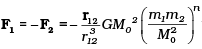where M0 is a constant of dimension of mass, r12 = r1 –r2 and n is a number. In such a case,
(a) The acceleration due to gravity on earth will be different for different objects
(b) None of the three laws of Kepler will be valid
(c) Only the third law will become invalid
(d) For n negative, an object lighter than water will sink in water
Ans.
(a, c, d)
Solution.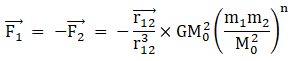Where M= constant
For calculating acceleration due to gravity let
M1 = Mass of earth = M
M2 = mass of object
r12 = radius of earth = R
Then magnitude of force between the object and earth is given by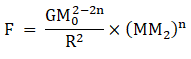Which can be said as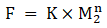Where K is a constant
Therefore, acceleration due to gravity can be calculated as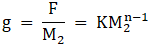Which depends on mass and hence different for different objects
Therefore (a) is the correct option
since this force follows inverse square law, hence Kepler’s first two are valid but since g is no longer constant, therefore Kepler’s third law (Law of periods) does not remain valid therefore (c) is the correct option
when n is negative than force is given by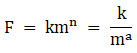Where a = - n
Therefore, force is inversely proportional to mass of body, hence an object lighter than water will experience more force than water and therefore will sink in water
Therefore (a), (c) and (d) are the correct options

Q.15 Which of the following are true?
(a) A polar satellite goes around the earth’s pole in north south direction
(b) A geostationary satellite goes around the earth in east-west direction
(c) A geostationary satellite goes around the earth in west-east direction
(d) A polar satellite goes around the earth in east-west direction
Ans.
(a, c)
Solution. The satellite which appears stationary relative to earth is called the geostationary satellite. It revolves around the earth in the west-east direction with the same angular velocity as done by the earth about its own axis in the west-east direction. A polar satellite revolves around the earth's pole in north-south direction. It is independent of earth's rotation. Option (a), (c) are correct.

Q.16 The centre of mass of an extended body on the surface of the earth and its centre of gravity
(a) Are always at the same point for any size of the body
(b) Are always at the same point only for spherical bodies
(c) Can never be at the same point
(d) Is close to each other for objects, say of sizes less than 100 m
(e) Both can change if the object is taken deep inside the earth
Ans.
(d)
Solution.
The center of gravity is based on weight, whereas the center of mass is based on mass. So, when the gravitational field across an object is uniform, the two are identical. However, when the object enters a spatially-varying gravitational field, the COG will move closer to regions of the object in a stronger field, whereas the COM is unmoved.
More practically, the COG is the point over which the object can be perfectly balanced; the net torque due to gravity about that point is zero. In contrast, the COM is the average location of the mass distribution or it is the point where whole mass of the body is supposed to be concentrated. If the object were given some angular momentum, it would spin about the COM.
For small objects, say of sizes less than 100 m placed in uniform gravitational field then centre of mass is very close with the centre of gravity of the body. But when the size of object increases, its weight changes and its CM and CG become far from each other. Like in the case of spherical ball, the CM and the CG are the same, but in case of Mount Everest, its CM lies a bit above its CG.

Q.1. Molecules in air in the atmosphere are attracted by gravitational force of the earth. Explain why all of them do not fall into the earth just like an apple falling from a tree.
Ans.
Air molecules in the atmosphere are attracted by gravitational force of the earth but they do not fall into earth due to the reason that the molecules in air has some random motion due to temperature, so their resultant motion is not exactly in the vertical downward direction.
But in case of apple, only vertical motion dominates because of being heavier than air molecules. But due to gravity, the density of air is more near to the earth than the density as we go up.

Q.2. Give one example each of central force and non-central force.
Ans.

Examples of central force:
(i) Gravitational force due to point mass.
(ii) Electrostatic force on the point charge.
Examples of non-central force:
(i) Nuclear force which depends upon the spin of particles.
(ii) Magnetic systems acting between two current carrying loops

Q.3. Draw areal velocity versus time graph for mars.
Ans.
Areal velocity of Mars revolving around the Sun does not change with time according to Kepler’s law, i.e. it is constant with time. Then graph of a real velocity versus time is a straight line parallel to time axis.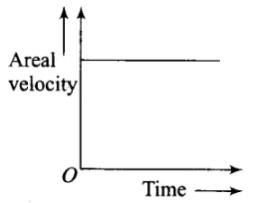Q.4. What is the direction of areal velocity of the earth around the sun?
Ans.
Areal velocity of the earth around the sun is given by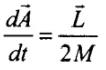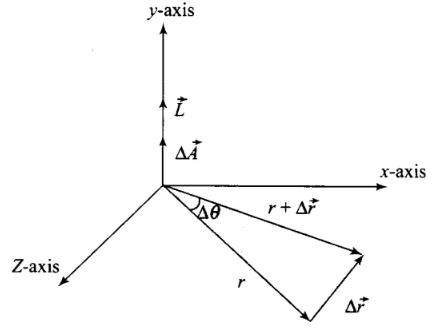where, L is the angular momentum and M is the mass of the earth.
But angular momentum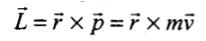Therefore we get,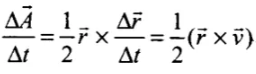Therefore, the direction of a real velocity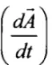is in the direction of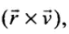i.e., perpendicular to the plane containing r and v and directed as given by right hand rule. So, areal velocity is normal to the plane containing Earth and Sun as shown in the figure

Q.5. How is the gravitational force between two point masses affected when they are dipped in water keeping the separation between them the same?
Ans.
Gravitational force acting between two point masses m1 and m2 is given by the relation,
F = G m1m2/ r2
It does not depend upon the medium separating the two point masses. Therefore, gravitational force acting between two point masses will remain unaffected when they are dipped in water keeping the separation between them same. Only their apparent weights change, there is no effect on masses.

Q.6. Is it possible for a body to have inertia but no weight?
Ans.
Yes, a body can have inertia but no weight. Inertia of a body is related to mass. Where as weight of body is defined as
W = mg
Where
M = mass of body
g = acceleration due to gravity
therefore, weight of the object can be zero when the acceleration due to gravity becomes zero.
Since we know that at the centre of earth, the value of acceleration due to gravity is zero and hence the weight of the object is zero at the centre, but it remains mass(inertia) remains constant.

Q.7. We can shield a charge from electric fields by putting it inside a hollow conductor. Can we shield a body from the gravitational influence of nearby matter by putting it inside a hollow sphere or by some other means?
Ans.
The gravitational force does not depend upon the nature of the medium, but the electric force depends on the intervening medium between the charge and the electric field. So we cannot shield a body from the gravitational force.

Q.8. An astronaut inside a small spaceship orbiting around the earth cannot detect gravity. If the space station orbiting around the earth has a large size, can he hope to detect gravity?
Ans.
Astronaut inside a small spaceship experience a very small negligible constant acceleration and hence astronaut feel weightlessness. If the space station has too much large mass and size then he can only experience acceleration due to gravity in the proximity of moon or on the moon.

Q.9. The gravitational force between a hollow spherical shell (of radius R and uniform density) and a point mass is F. Show the nature of F vs r graph where r is the distance of the point from the centre of the hollow spherical shell of uniform density.
Ans.

Given:
Radius of hollow shell = R
Distance of point mass from centre of hollow shell = r
Let the mass of the spherical shell be M and the point mass be m. Then the force between the shell and the point mass is given by,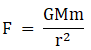When point mass is positioned such that it is inside the hollow sphere i.e. 0<r<R, the force is zero as the mass of the hollow sphere is distributed on its surface. When the mass is positioned such that r≤R, the force will be,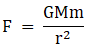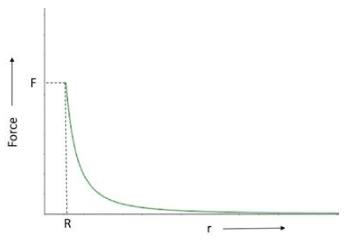Therefore, the graph will be as follows

Q.10. Out of aphelion and perihelion, where is the speed of the earth more and why ?
Ans.
Aphelion is the location of earth from where it has the greatest distance from the sun and perihelion is the position from where it has the shortest distance. Now since the angular momentum of earth about the sun is conserved then we have:
mrap vap = mrpevpe
Where m is the mass of the earth, rap is the earth - sun distance at the aphelion and rpe is the earth - sun distance at the perihelion.
∴ rapvap = rpevpe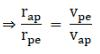Since rap > rpe, vpe > vap, therefore the velocity at the perihelion is greater than the velocity at the aphelion.

Q.11. What is the angle between the equatorial plane and the orbital plane of
(a) Polar satellite?
(b) Geostationary satellite?
Ans.
The orbital plane of a satellite is the plane in which it revolves around the earth. The equatorial plane of earth is the plane which contains the equator.
(a) For a polar satellite, the satellite revolves from north to south and therefore its orbital plane is at 90° to the equatorial plane
(b) For a geostationary satellite whose position with respect to a place on earth is constant i.e. it revolves in the direction of the rotation of earth, the orbital plane is at 0° to the equatorial plane.

Offer running on EduRev: Apply code STAYHOME200 to get INR 200 off on our premium plan EduRev Infinity!

,

,

,

,

,

,

,

,

,

,

,

,

,

,

,

,

,

,

,

,

,

;